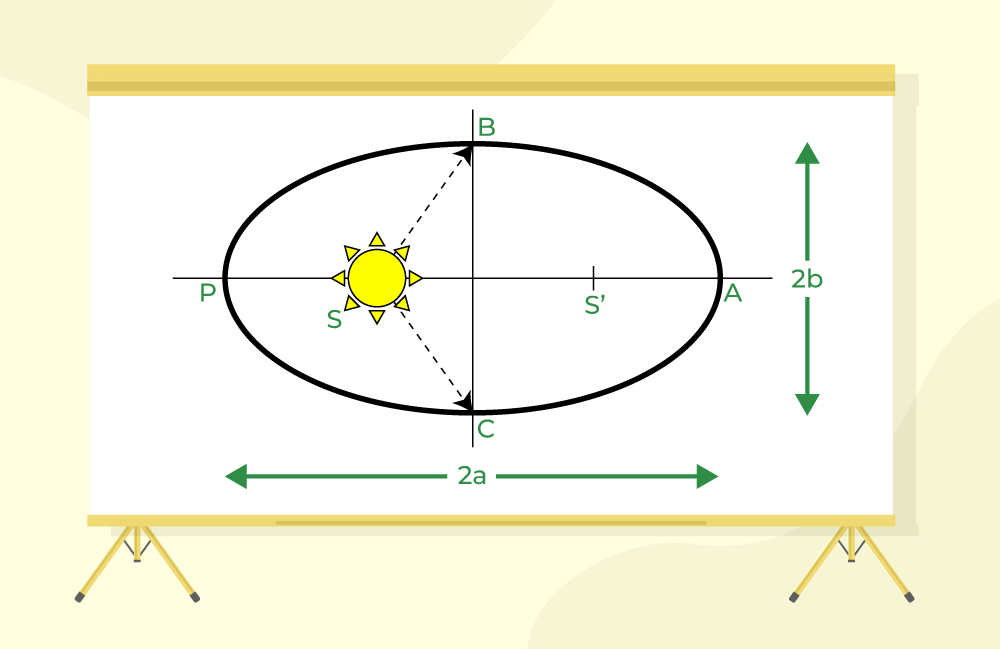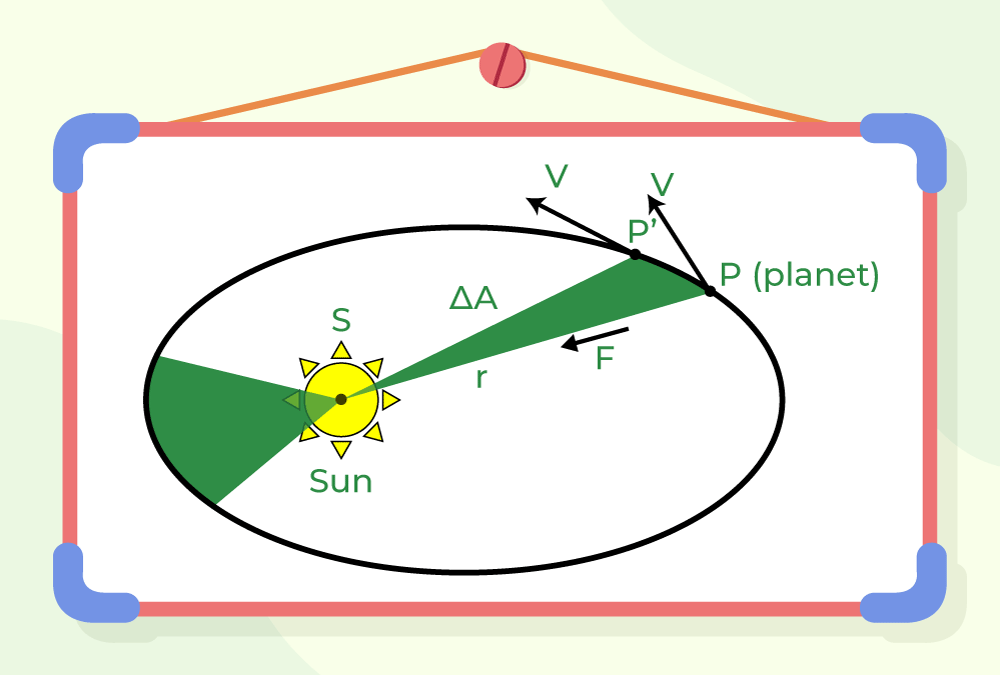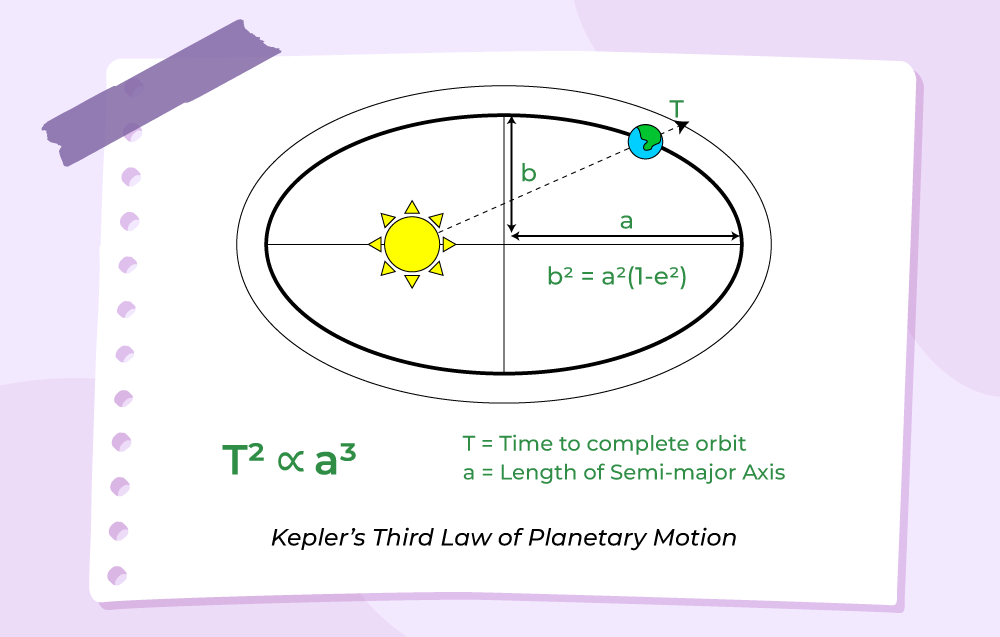GFG App
Open AppBrowser
Continue

# Kepler’s Laws of Planetary Motion

Kepler’s law of planetary motion is the basic law that is used to define the motion of planets around the stars. These laws work in parallel with Newton’s Law and Gravitation Law and are helpful in studying the motion of various planetary objects.

Kepeler’s law provides three basic laws which are,

• Kepler’s First law (Law of Orbits)
• Kepler’s Second law (Law of Areas)
• Kepler’s Third law (Law of Periods)

These laws are provided by the German astronomer Johannes Kepler, hence the name Kepeler’s Law. Let’s learn about these in detail in this article.

## Introduction to Kepler’s Law

The motion of an object is always relative with respect to other motions. Motions are divided into two categories based on the energy of the particle in motion:

• Bounded Motion
• Unbounded Motion

Bounded Motion

The particle possesses negative total energy (E < 0) in bounded motion and has two or more extreme points where the total energy is always equal to the particle’s potential energy, i.e. the particle’s kinetic energy becomes zero.

When the eccentricity 0 ≤ e < 1, E < 0 denotes that the body’s motion is bounded. The eccentricity of a circular orbit is zero, whereas the eccentricity of an elliptical orbit is one.

Unbounded Motion

The particle in unbounded motion has positive total energy (E > 0) and a single extreme point where the total energy is always equal to the particle’s potential energy, i.e. the particle’s kinetic energy becomes zero.

When the eccentricity e ≥ 1, E > 0 denotes that the body is moving freely. The eccentricity of a parabolic orbit e = 1, while the eccentricity of a hyperbolic orbit e > 1.

Law of orbits, the law of equal areas, and the law of periods are Kepler’s first, second, and third laws described in detail below.

## Kepler’s First Law: The Law of Orbits

Kepler’s First Law known as the law of ellipses, states that planets revolve around the sun in an elliptical pattern. The law of orbits is a common name for Kepler’s first law.

According to the rule, any planet’s orbit is an ellipse around the Sun, with the Sun at one of the ellipse’s two focal points.

Planets circle the Sun in a circular orbit, which we are already familiar with. Planets do, however, rotate around the Sun, although not in a circular orbit, according to Kepler. However, it is centered on an ellipse.There are two foci (S & S’) in an ellipse. The sun (S) is located at one of the ellipse’s focal points. Perihelion (PS) refers to the point when the planet is closest to the sun, whereas aphelion (AS) refers to the point where the planet is furthest from the sun. The major axis is (2a) and the minor axis is (2b). The total of any planet’s distances from two foci is constant, which is one of the properties of an ellipse. Seasons are brought about by a planet’s elliptical orbit.

## Kepler’s Second Law: The Law of Areas

Kepler’s Second Law, often known as the law of areas, determines the rate at which each planet will spin while circling the sun. The rate at which each planet rotates through space is continuously changing. When a planet is closest to the sun, it spins the quickest, and when it is furthest from the sun, it revolves the slowest.

According to Kepler’s second rule, when the orbiting satellite approaches the focus, it will accelerate. As the orbiting item comes closer to the object pushing it into the orbital path, the gravitational impact on it increases.

The area covered by the planet or spinning object in giving time is the same, regardless of the distance to the item at the focus, according to the mathematical formulation of the law. Because the areas are equivalent, the arc that is further away is shorter, resulting in a slower speed. This holds true for all objects in orbit.The regions developed when the earth is nearest to the sun can be calculated as a broad but short triangle, as shown in the picture below. The regions formed when the earth is furthest from the sun, on the other hand, can be approximated as a narrow but long triangle. These regions are around the same size. When the earth is farthest from the sun, the base of these triangles is the shortest. It has been noticed that for this hypothetical region to have the same size as when the earth is closest to the sun, the earth would have to move more slowly when it is farthest from the Sun.

Kepler’s Second Law: (Law of Areas) has its relation to the Law of Conservation of Momentum so first learn about it in detail.

### Law of Conservation of Momentum

This law can also be verified by the law of conservation of angular momentum which states that the momentum of a planet revolving around the star in a fixed orbit is always constant along the orbit.

Angular momentum of the planet at position B, LB = m rB vB.

Angular momentum of the planet at position D,  LD = m rD vD.

Now, since L is constant at any point, so LB = LD

rB vB = rD vD

The above expression shows that the distance and speed are inversely related to each other for a planet revolving in an orbit.

Area enclosed by a planet in an orbit is given by,

dA ⁄ dt = L ⁄ 2 m

where
dA is the area enclosed by a planet
dt is the time taken to enclose the area
m is the mass of the planet
L is the angular momentum of the planet

## Kepler’s Third Law: The Law of Periods

Kepler’s Third Law, often known as the law of periods, establishes a comparison of a planet’s orbital period and radius of orbit to those of other planets. The 3rd law compares the motion characteristics of various planets, as opposed to Kepler’s 1st and 2nd laws, which describe the motion property of a single planet.

Kepler’s third law defines the relationship between the mass of the planet star system, the distance of planets from the star, and their orbital period. It states that the square of the time period of a planet is directly proportional to the cube of the semi-major axis of the orbit of the planet and inversely proportional to the sum of the mass of the star and planet.The formula for Kepler’s third law is stated as:

T2 ∝  a3

T2 = (4 π2 a3) ⁄ [G (M + m)]

where
T is the time period
M is the mass of Sun
m is the mass of planet
R is the length of semi-major axis
G is the gravitational constant

## Newton’s Laws and Kepler’s Laws

The authenticity of Kepler’s Laws is implied by Newton’s Laws

According to the first law of motion, an object accelerates only when a net force is applied which is in compliance with Kepler’s first Law which implies planets revolve around the sun in elliptical orbits with the Sun at one of its foci.

Also, the planet’s areal speed is constant which is in accordance with Kepler’s 2nd Law i.e. planets cover an equal area in equal time intervals.

Both Kepler’s 3rd Law and Newton’s 3rd Law imply that the force acting on the planet is proportional to the product of the masses of the planet and the Sun.

Thus, all three laws of Newton’s are in compliance with Kepler’s Law of Planetary motion.

## Solved Examples on Kepler’s law

Example 1: The radius of a planet is 3 times the radius of the Earth. Calculate the time period of the revolution of the planet.

Solution:

According to Kepler’s third law:

T2 ∝ R3

Consider the radius of the Earth be Re, radius of the planet be Rp, time period of the Earth be Te and time period of the planet be Tp.

Given:

Rp = 3 Re

Time period of the Earth, Te = 1 yr

The relation between the planets is given as:

Tp2 ⁄ Te2 = Rp3 ⁄ Re3

Tp2 ⁄ (1 yr)2 = (3 Re)3 ⁄ Re3

Tp2 = 27 yr

Tp = 5.2 yr

Hence, the time period of the planet is 5.2 years.

Example 2: The radius of a planet is 8 times the radius of the Earth. Calculate the time period of the revolution of the planet.

Solution:

According to Kepler’s third law:

T2 ∝ R3

Consider the radius of the Earth be Re, radius of the planet be Rp, time period of the Earth be Te and time period of the planet be Tp.

Given:

Rp = 8 Re

Time period of the Earth, Te = 1 yr

The relation between the planets is given as:

Tp2 ⁄ Te2 = Rp3 ⁄ Re3

Tp2 ⁄ (1 yr)2 = (8 Re)3 ⁄ Re3

Tp2 = 512 yr

Tp = 22.62 yr

Hence, the time period of the planet is 22.62 years.

Example 3: The ratio of orbital angular momentum (about the sun) to the mass of the Earth is 4.4 × 1015 m2 ⁄ s. Find the area enclosed by the Earth.

Solution:

Given:

L ⁄ m = 4.4 × 1015 m2 ⁄ s

Time taken to cover the complete orbit is equal to the time period of the Earth,i.e.,

dt = 365 days

= 365 × 86400 s

= 31536000 s

Area enclosed by a planet in an orbit is given by:

dA ⁄ dt = L ⁄ 2 m

dA = (L ⁄ m) × (dt ⁄ 2)

= (4.4 × 1015 × 31536000) m2

= 6.938 × 1022 m2

Hence, the area enclosed by the Earth is equal to 6.938 × 1022 m2.

## FAQs on Kepler’s law

### Question 1:  How did Newton extend his notion of Gravity to Explain Planetary Motion?

Newton’s First law is an inverse square law, i.e. it says that the force between two objects is inversely proportional to the square of their distances whereas, Kepler’s 1st Law states that the planets revolve around the sun in elliptical orbits with Sun at one focus. The two law support each other in defining the motion of the planets.

### Question 2: How Kepler’s First Law describes the Shape of an Orbit?

Kepler’s First Law also called Law of orbits, states that planets revolve around the sunfollowing an elliptical path and sun is at any of the focus of the ellipse.

### Question 3: How Kepler’s Second Law describes the way a planet’s speed varies along its orbit?

According to Kepler’s Second Law of Planetry motion, the areal speed of a planet is always constant. So it the planet is closer to sun it accelerates at faster velocity and if the planet is away from sun it accelerates slowly.